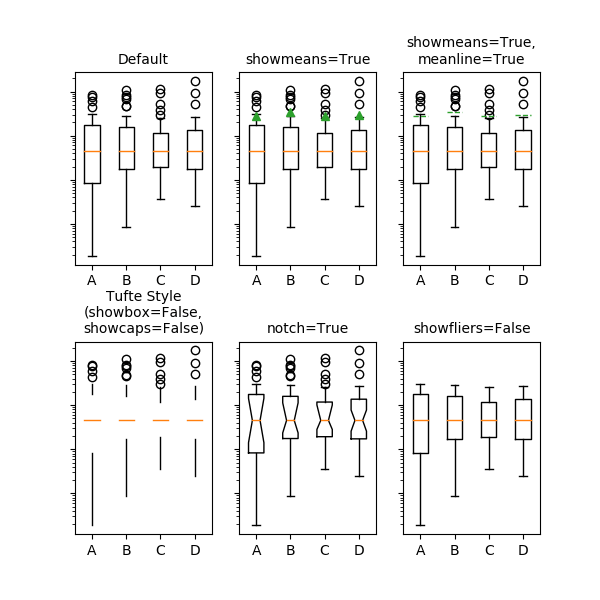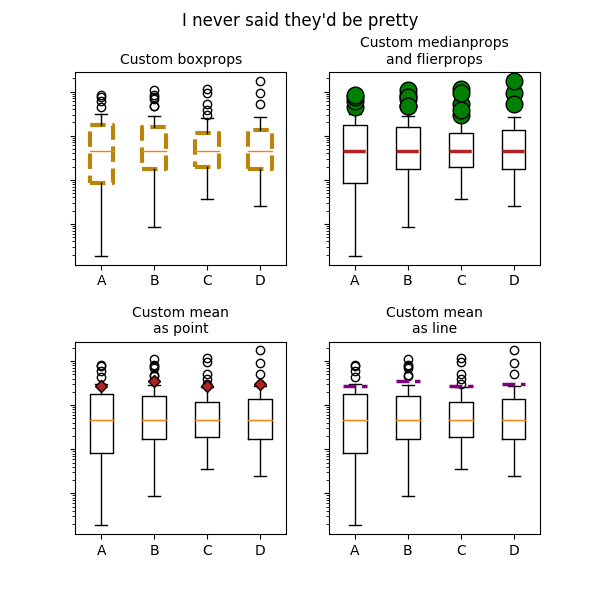/Matplotlib 3.1

# matplotlib.axes.Axes.bxp

`Axes.bxp(self, bxpstats, positions=None, widths=None, vert=True, patch_artist=False, shownotches=False, showmeans=False, showcaps=True, showbox=True, showfliers=True, boxprops=None, whiskerprops=None, flierprops=None, medianprops=None, capprops=None, meanprops=None, meanline=False, manage_ticks=True, zorder=None)` [source]

Drawing function for box and whisker plots.

Make a box and whisker plot for each column of x or each vector in sequence x. The box extends from the lower to upper quartile values of the data, with a line at the median. The whiskers extend from the box to show the range of the data. Flier points are those past the end of the whiskers.

Parameters: `bxpstats : list of dicts` A list of dictionaries containing stats for each boxplot. Required keys are: `med`: The median (scalar float). `q1`: The first quartile (25th percentile) (scalar float). `q3`: The third quartile (75th percentile) (scalar float). `whislo`: Lower bound of the lower whisker (scalar float). `whishi`: Upper bound of the upper whisker (scalar float). Optional keys are: `mean`: The mean (scalar float). Needed if `showmeans=True`. `fliers`: Data beyond the whiskers (sequence of floats). Needed if `showfliers=True`. `cilo` & `cihi`: Lower and upper confidence intervals about the median. Needed if `shownotches=True`. `label`: Name of the dataset (string). If available, this will be used a tick label for the boxplot `positions : array-like, default = [1, 2, ..., n]` Sets the positions of the boxes. The ticks and limits are automatically set to match the positions. `widths : array-like, default = None` Either a scalar or a vector and sets the width of each box. The default is ```0.15*(distance between extreme positions)```, clipped to no less than 0.15 and no more than 0.5. `vert : bool, default = True` If `True` (default), makes the boxes vertical. If `False`, makes horizontal boxes. `patch_artist : bool, default = False` If `False` produces boxes with the `Line2D` artist. If `True` produces boxes with the `Patch` artist. `shownotches : bool, default = False` If `False` (default), produces a rectangular box plot. If `True`, will produce a notched box plot `showmeans : bool, default = False` If `True`, will toggle on the rendering of the means `showcaps : bool, default = True` If `True`, will toggle on the rendering of the caps `showbox : bool, default = True` If `True`, will toggle on the rendering of the box `showfliers : bool, default = True` If `True`, will toggle on the rendering of the fliers `boxprops : dict or None (default)` If provided, will set the plotting style of the boxes `whiskerprops : dict or None (default)` If provided, will set the plotting style of the whiskers `capprops : dict or None (default)` If provided, will set the plotting style of the caps `flierprops : dict or None (default)` If provided will set the plotting style of the fliers `medianprops : dict or None (default)` If provided, will set the plotting style of the medians `meanprops : dict or None (default)` If provided, will set the plotting style of the means `meanline : bool, default = False` If `True` (and showmeans is `True`), will try to render the mean as a line spanning the full width of the box according to meanprops. Not recommended if shownotches is also True. Otherwise, means will be shown as points. `manage_ticks : bool, default = True` If True, the tick locations and labels will be adjusted to match the boxplot positions. `zorder : scalar, default = None` The zorder of the resulting boxplot. `result : dict` A dictionary mapping each component of the boxplot to a list of the `matplotlib.lines.Line2D` instances created. That dictionary has the following keys (assuming vertical boxplots): `boxes`: the main body of the boxplot showing the quartiles and the median's confidence intervals if enabled. `medians`: horizontal lines at the median of each box. `whiskers`: the vertical lines extending to the most extreme, non-outlier data points. `caps`: the horizontal lines at the ends of the whiskers. `fliers`: points representing data that extend beyond the whiskers (fliers). `means`: points or lines representing the means.

#### Examples(png, pdf)